# Multi-objective Optimization for a Complex Intersection Using Design of Experiments and Simulation

Multi-objective Optimization for a Complex Intersection Using Design of Experiments and Simulation
Department of Industrial Engineering, Esfarayen University of Technology, North Khorasan 96619-98195, Iran

Corresponding Author Email:
a.ghorbanian@esfarayen.ac.ir
Page:
791-802
|
DOI:
https://doi.org/10.18280/jesa.530605
20 August 2020
|
Accepted:
26 November 2020
|
Published:
23 December 2020
| Citation

OPEN ACCESS

Abstract:

Considering the importance of time in today's world and the rising traffic congestion in urban areas, using methods to reduce wait times and air pollution can have a significant impact on promoting urban management. Given the uncertainty in the number of vehicles and the emission rate of vehicles, a complex T intersection with three traffic lights was simulated in this study. Three objective functions were defined for the mean of wait time, average queue length, and aggregate pollutant emission of the vehicles in queue. First, regression equations for each of the variables were obtained by a full factorial design and analysis of variance, and the optimal period for each traffic light was then computed with a utility function approach. Finally, the results were compared to the results obtained from the optimization of each response variable OptQuest for Arena software.

Keywords:

traffic in urban areas, simulation, multi-objective, design of experiments

1. Introduction

In the twentieth century, a large number of vehicles and population increase has led to a decrease in the effectiveness of urban passageways. The improvement of transport networks is largely limited by the boundary of the existing networks . Nowadays, cities have to deal with traffic congestion caused by an increase in the number of vehicles and the need for transportation . Solving problems such as traffic congestion, air pollution, and fuel waste, which are caused by traffic, are of great importance . Fuel consumption is also a significant parameter; for example, in the United States drivers spend 40 hours per year stuck in traffic. The cost of the fuel that is used in these 40 hours is about 87 billion dollars per year . Pollution is also one of the permanent problems in today's world . A study has been conducted on bus drivers to detect the probable damages to DNA. Traffic congestion has adverse effects on human health, especially in big cities . In industrialized countries, and especially in major cities, air pollution is one of the factors that affect the population and is associated with many diseases such as cancer [7, 8]. Another issue is the time people waste in traffic. An origin and destination study were conducted to investigate this wasted time . Simulation is one of the most common methods for analyzing and solving problems in a complex transport network . Bieker et al.  presented a scenario for Bologna's traffic that could be used as a source for more research. The semantic language of the Web is studied as well . The amount of deviation from molecules’ mechanical force is also examined to simulate the structure . Five software programs were used to evaluate the simulation of several models . A multi-agent simulation environment that could be generalized for Java programming is presented . Balci  presented a large-scale model to determine the quality of the application of modeling and simulation. Simulation is also of importance in health and medicine. Simulating a Glucose-insulin system, which was done by Dalla et al. , can greatly help diabetic patients. Analysis of different approaches and evaluation of their effects in a clear and comprehensible way is also possible through simulation. Selecting the right software is an important issue as well. Among various software programs, a great number of researchers have used Arena; for instance, this software was used to simulate the design and analysis of a coastal town . Bush et al. presented a technique that utilizes both optimization and simulation . An automated driving system for vehicles is simulated with regard to JIT philosophy by Kesen et al. . Arena Software is used for reliability and simulation in health systems . One of the most common uses of Arena is in modeling different types of transportation. Almaz et al. have provided a model for Vessel Traffic . The simulation of the transportation of cargo in Sevia, a port city, is also noteworthy . Arena has been used for the simulation of the Newcastle railway network and to show the efficiency of railways . Through simulation modeling in Arena, the amount of use of various parts of the railway was computed . Due to time change, fuzziness, and non-linearity, artificial intelligence is used in traffic control . D’Ambrogio et al. have presented a method to help designers simulate traffic systems . The traffic flow in a T-shaped intersection is simulated using Monte Carlo techniques . Arena software is also used to simulate a T-shaped intersection , the intersection is then studied in two different situations: with and without traffic lights. The results were encouraging; thus, Arena can be used as a tool to model various transportation issues and find a solution for the problems. Arena is used to model a traffic signal system . As mentioned before, simulation is done to model many types of traffic and analyze the solutions. Since Arena is easily available for researchers, the present study has selected this software for simulation. The comprehensiveness of Arena also lends to its use for purposes other than production, which was another reason that we decided to use this software.

The literature review reveals that most articles only study queues at intersections and are not concerned with issues such as pollution. They also merely use mathematical models and as a result, uncertainty factors are investigated less. Therefore, the present study attempts to use these two methods simultaneously. Thus, parameters such as entry rate for each of intersection entrances and the amount of emission per vehicle are regarded as probable variables. The amount of emission for various vehicle types is also separately considered. Figure 1 shows a schematic abstract of the problem.

In this study, we have simulated a T-shaped intersection in the city of Bojnourd, using Arena software. Intersections are one of the most common areas in which traffic congestion happens. As mentioned, many solutions to reduce traffic at crossroads and intersections have been proposed, but simulation and the analysis of simulated conditions can provide very useful information with minimal cost and trial and error. These two features of simulation distinguish it and make it very important in transportation issues, as these issues are quite complex and costly. The simulation gives us a systematic view, which can help provide new solutions.

This paper is organized as follows. We present a description of the Proposed Approach, Selected methods, and evaluation metrics used to compare the algorithms in Section 2. Section 3 presents three mathematical models. Section 4 concludes the simulation model and results. Section 4 presents the design of experiment (DOE) and regression Equations for optimization. Section 6 concludes the Optimization approach for desirability function as well as discussing the advantages and disadvantages of the selected methods. The main contribution is the optimization of three objectives function average wait time, the average number of vehicles in the queue, and total Pollutant emission simultaneous by using experiment design and simulation-based on desirability functions.

## 1.png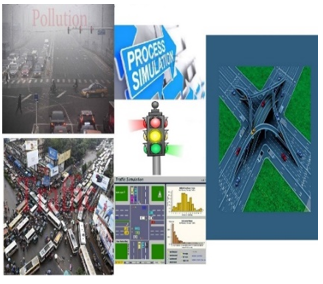Figure 1. Schematic abstract

2. The Proposed Approach

The present study attempts to provide an optimal approach for finding the best time intervals for traffic lights. As all events in an intersection have probable times and uncertainty, using a mathematical model is difficult, as a result, we have used a simulation to investigate the contributing factors. A complex intersection with a 3-phase traffic light was selected for simulation.

## 2.pngFigure 2. The proposed approach

Given that all the events that happened at an intersection are uncertain in terms of time, using a mathematical model is rather difficult. Hence, a simulation model is used for different factors. In this study, a complex intersection with three-phase traffic lights is simulated. As shown in Figure 2, first we defined a mathematical model for the objective functions. Because of the probability of parameters, the simulation was used. The central points of the response variables were studied by the use of a two-stage full factorial design, and for each of the objective functions, a mathematical regression model was provided. Next, a desirability function was used to find the optimal response for each of the three response variables. Ultimately, the obtained responses were used as initial responses to optimize each of the objective functions in OptQuest software and the results were analyzed.

OptQuest is a generic optimizer in ARENA software that makes it possible to separate successfully the optimization solution procedure from the simulation model. This design adaptation of meta-heuristic methods lets you create a model of your system that includes as many elements as necessary to represent the “real thing” accurately. While the simulation model can change and evolve to incorporate additional elements, the optimization routines remain the same. Hence, there is a complete separation of the model that represents the system and the procedure that solves optimization problems defined within this model, which provides maximum efficiency in identifying new scenarios. the advantages of OptQuest are Efficient, Intelligent, Flexible, and Aggressive.

3. Mathematical Model and Objective Functions

To optimize the model, a mathematical model is presented. This model has three objective functions. The first objective is to minimize the average wait time. Minimizing the average number of vehicles in the queue is also very important. Pollutant emission is also the third objective in this model.

\begin{aligned} T W T=M W T a_{1} &+M W T a_{2}+M W T b_{1} \\ &+M W T b_{2}+M W T b_{3}+M W T c_{2} \\ &+M W T c_{3} \end{aligned}    (1)

\begin{aligned} T N Q=M N Q a_{1} &+M N Q a_{2}+M N Q b_{1} \\ &+M N Q b_{2}+M N Q b_{3}+M N Q c_{2} \\ &+M N Q c_{3} \end{aligned}    (2)

$T E=E a_{1}+E a_{2}+E b_{1}+E b_{2}+E b_{3}+E c_{2}+E c_{3}$    (3)

$E a_{1}=\sum_{i=1}^{n_{a}} r_{i} w t_{i}$    (4)

Eq. (1) shows the mean of wait time for all intersections, which is obtained from the sum of all means of wait times in different lines. Eq. (2) shows the mean the number of cars waiting in the queue, which is the sum of means in different lines. Eq. (3) shows the total amount of emissions created by vehicles. Eq. (4) shows the aggregate pollutant emission of the vehicles in the queue, which is the sum of emissions by each vehicle. The amount of emission of each vehicle is obtained by multiplying the vehicle wait time by its emission rate. To obtain the amount of emission of each vehicle, we used the Euro 4 standard, which is the standard currently used in Iran. Table 1 shows the amount of emission for each vehicle per minute in four main categories based on European emission standards .

Table 1. Emission rate by Euro 4 Standard

 Row Date CO NOx HC+NOx PM g/Km g/min Category 1 Jan-05 0.5 0.25 0.3 0.03 1.43 1.075 Category 2 Jan-05 0.5 0.25 0.3 0.03 1.43 1.075 Category 3 Jan-06 0.63 0.33 0.39 0.04 1.85 1.39 Category 4 Jan-06 0.74 0.39 0.46 0.06 2.20 1.65
4. Simulation

The following set of hypotheses were considered in our simulation.

1. No accident or breakdowns results in a stop.

2. The cars that are going towards the exits will not get in any queues.

3. The cars in the queue of the last line of C will only move into B, and the car in the queue of the middle line of C will only move into A (those who want to follow a straight line in C will move on through the first line of C, without getting into any queues).

4. If the cars wait more than 90 seconds, 90% of the drivers will turn their cars off to reduce emissions.

5. The four types of vehicles entering the intersection are in accordance with the Euro standard.

Figure 3 shows a schematic plan of the intersection. Based on the above-mentioned explanations and a real model, the present study presents a simulation model generated by Arena software, as shown in Figure 4. Each of the modules used in the model is discussed as follows.

## 3.png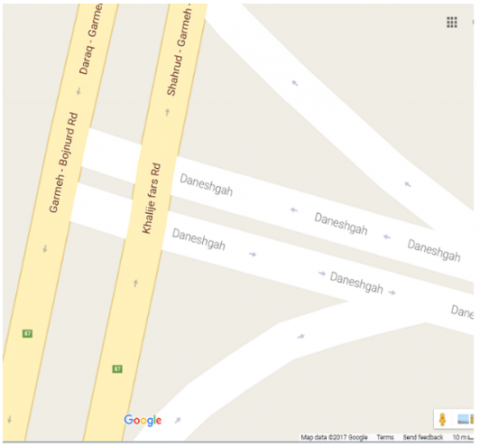Figure 3. Schematic of the simulated location

## 4.pngFigure 4. Simulated model

Figure 4 shows the model which is simulated for the intersection. It consists of four main parts: three of which (A, B, and C) are the main intersection entrances and the other one is the time length of each traffic light. Each one of the entrance simulation models has a sub-model that Appendix1 shows it.

4.1 Warm-up

Considering that the results of a simulation cannot be used until the model reaches a stable state, obtaining the WU time is very important. As such, a graph (Figure 5) is used to show the approximate time of reaching a stable state. The horizontal index presents the time and the vertical index, the mean the number of vehicles in queue. As shown, the stable state happens after about 40 minutes. This period is considered as WU.

## 5.pngFigure 5. Warm up period

5. Experiments Design

The main purpose of the design of experiment (DOE) is to obtain the maximum amount of data with a minimum number of experiments

5.1 Obtaining high and low levels

To obtain the high and low levels for each decision, Opt Quest software was used for each decision variable. To this purpose, we assumed that the objective of the whole simulation is to minimize the wait time for each of the decision variables. To minimize the mean, wait time, the decision variable must be at its highest value. The value of -1 was assigned for the low level of each decision variable; thus, -1 is the minimum time required for a vehicle to cross the intersection. The high and low levels for each of the decision variables are shown in Table 2.

Table 2. High and low levels of the variables

 High level Low level Time a 260 9 Time b 400 9 Time c 470 9

5.2 Design of experiments

To do the experiments, a full-factorial design was used with 3 decision variables. The experiment was simulated four times for the central points and each of the corners, and the results are shown in Tables 3, 4, and 5.

Table 3. Results of simulations for design of experiments for mean of wait time

 time a time b time c Total waiting time(min) Replication1 Replication2 Replication3 Replication4 -1 -1 -1 3.1707 3.1523 3.6739 3.1721 1 -1 -1 1,937.72 1,939.26 1,954.31 1,933.73 -1 1 -1 1,543.39 1,568.49 1,555.31 1,552.42 1 1 -1 859.01 854.68 846.05 855.80 -1 -1 1 1,963.95 1,952.34 1972.21 1,950.94 1 -1 1 1,249.96 1,247.40 1,259.39 1,247.63 -1 1 1 831.14 831.11 826.09 824.11 1 1 1 39.9175 42.7596 48.1784 46.0691 0 0 0 24.0286 30.3630 29.7507 23.3526

Table 4. Results of simulation for design of experiments for mean of number of vehicles in queue

 time a time b time c Total number in queue Replication1 Replication2 Replication3 Replication4 -1 -1 -1 9.2097 10.1411 9.4696 10.9055 1 -1 -1 7,967.61 8,131.74 8,082.71 8,007.59 -1 1 -1 5,815.35 5,927.18 5,937.46 5,929.42 1 1 -1 4,387.88 4,432.48 4,464.65 4,477.32 -1 -1 1 5,827.67 5,826.43 5,864.84 5,755.47 1 -1 1 4,324.84 4,387.62 4,313.66 4,492.83 -1 1 1 1,788.16 1,872.16 1,863.22 1,819.59 1 1 1 82.4994 102.42 120.87 134.01 0 0 0 52.5650 106.57 60.9502 71.9519

Table 5. Results of simulation for design of experiments for the aggregate emission

 time a time b time c Total emission(gram) Replication1 Replication2 Replication3 Replication4 -1 -1 -1 4,157.83 4,259.82 4,147.35 4,214.76 1 -1 -1 33,341.03 28,182.85 33,295.75 30,727.49 -1 1 -1 20,797.25 16,121.83 20,700.04 14,442.45 1 1 -1 7,790.56 10,790.15 8,074.65 10,927.50 -1 -1 1 17,726.63 17,665.41 17,041.98 18,356.61 1 -1 1 12,518.55 9,301.62 8,411.55 10,190.79 -1 1 1 7,255.96 8,081.18 9,187.53 6,076.61 1 1 1 5,085.93 7,275.59 6,025.86 5,319.77 0 0 0 4,018.05 4,175.02 4,135.41 4,041.14

5.3 Results of design of experiments

To verify the design variables, a two-stage full factorial design with central points is used. Table 6 shows the results of the three objective functions.

Table 6. Analysis of variance total emission total number in queue total waiting time

 total emission Total number in queue total waiting time Coef P-Value Coef P-Value Coef P-Value Constant 1054.58 0.000 3818.04 0.000 13047 0.000 time a -31.96 0.000 426.38 0.000 1157 0.001 time b -234.30 0.000 -745.88 0.000 -2800 0.000 time c -33.75 0.000 -782.03 0.000 -2702 0.000 time a*time b -339.26 0.000 -1223.28 0.000 -3743 0.000 time a*time c -341.20 0.000 -1217.55 0.000 -3486 0.017 time b*time -350.36 0.000 -1317.27 0.000 -757 0.000 time a*time b*time c 320.48 0.000 1151.54 0.000 5210 0.000

## 6a.png## 6b.png## 6c.pngFigure 6. Distribution of errors

5.4 Normality test

The normal graph is drawn for each of the errors (Figure 6).

As the images show, the errors are approximately on a straight line in each case. This indicates the normality of errors for any of the response values. Statistical tests could also be used to examine the claims.

5.5 Dispersion index

## 7a.png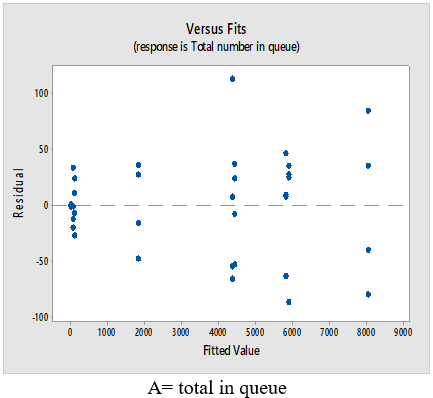## 7b.png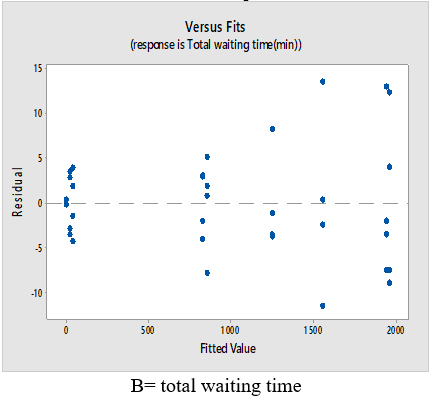## 7c.pngFigure 7. Dispersion of errors

Another index to take into account is the equality of distribution of errors for each of the stages. As shown in Figure 7, a slight difference in dispersion levels can only be seen for the emission response. However, these values are not considerable and the distribution in each stage is approximately equal for the other two responses, and there are no outliner data.

5.6 Correlation

## 8a.png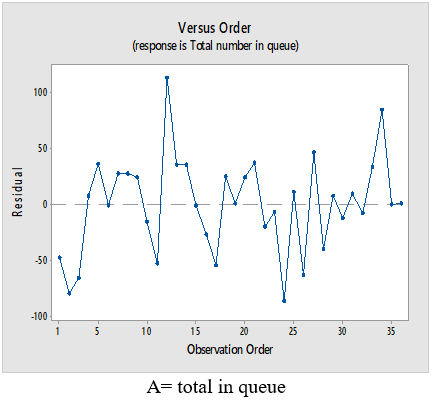## 8b.png## 8c.pngFigure 8. Correlation errors for each response variable

The lack of correlation or any specific relations in the data should also be considered. As shown in Figure 8, the data is distributed randomly, and there is no special correlation for any of the responses.

As each of the response variables has the required features to use the experiment design and regression lines, we can use the obtained results. To investigate the decision variables, a two-stage full factorial design with central points is applied. The results for the three objective functions are shown in Table 6.

5.7 Analyzing the effect of each factor on response variables

Figure 9 shows the main as well as the interaction effect of each factor on the response variable of the number of vehicles in the queue. There is an interaction between each of the three decision variables. Considering the main effect of each factor in minimizing the number of cars in queue, the number of cars in time A should be at a low level, and time B and C, at a high level.

Figure 10 shows the main and interaction effect of each decision variable on the mean of wait time. As seen, interaction is present in all factors in the response. The decision variables A and C have an insignificant effect and the responses in various levels are not much different.

## 9a.png## 9b.png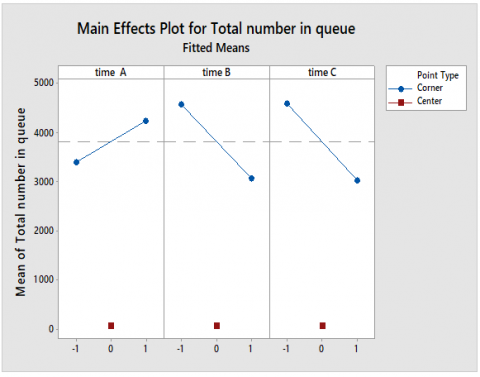Figure 9. Interaction effect of the decision variables on response variable of number of cars in queue

## 10a.png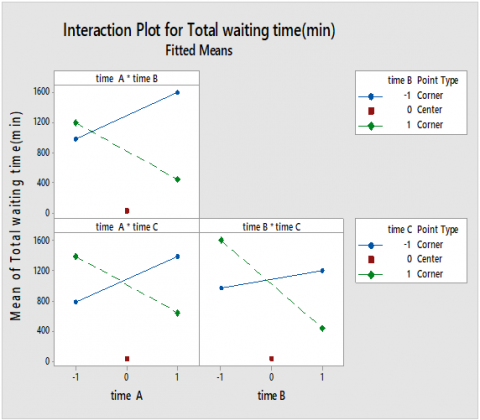## 10b.pngFigure 10. Interaction effect of the decision variables on response variable of mean of time

Figure 11 shows the main and interaction effects of decision variables on the response variable of the amount of emission. As seen, the decision variables A and B, as well as A and C do not have a significant interaction effect.

## 11a.png## 11b.png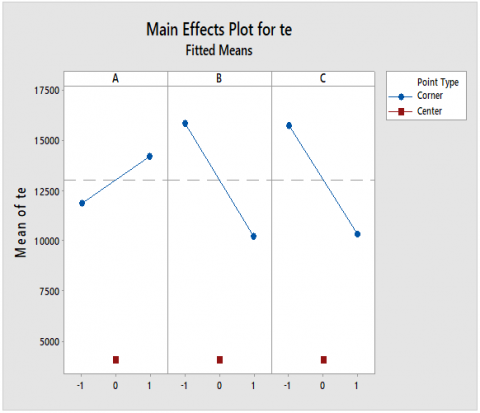Figure 11. Interaction effect of the decision variables on response variable of total emission

Figure 12 shows the factors affecting each of the response variables. All of the decision variables and their interaction effect on each other have a main effect on all of the response variables.

## 12a.png## 12b.png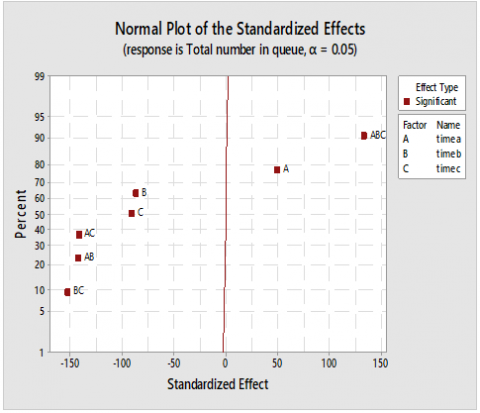## 12c.pngFigure 12. Factors affecting each response variable

5.8 Regression equations

A regression model is used to predict each of the objective functions. The regression model consists of a first-order regression and the interaction effect between them .

A general model for the first-order regression with three factors and the relationship between the factors is shown in the Eq. (5).

$D=\beta_{0}+\sum_{i=1}^{3} \beta_{i} x_{i}+\sum_{i=1, i<j}^{3} \beta_{i j} x_{i} x_{j}+\epsilon$    (5)

Based on the results of ANOVA and the design of experiments, a regression equation is defined for each of the objective functions. The regression equation of the mean of total waiting time is shown in Eq. (6) and the mean of total vehicles in the queue is shown in Eq. (7). The total amount of emission is shown in Eq. (8).

\begin{aligned} T W T=1054.58 &-31.96 \times a-234.30 \times b \\ &-33.75 \times \mathrm{c}-339.26 \times \mathrm{a} \times \mathrm{b} \\ &-341.20 \times \mathrm{a} \times \mathrm{c} \\ &-350.36 \times \mathrm{b} \times \mathrm{c} \\ &+320.48 \times \mathrm{a} \times \mathrm{b} \times \mathrm{c} \end{aligned}    (6)

$T N Q=3818.04+426.38 \times a-745.88 \times b$

$-782.03 \times c$

$-1223.28 \times a \times b$

$-1217.55 \times a \times c$

$-1317.27 \times b \times c$

$+1151.54 \times a \times b \times c$    (7)

$T E=13047+1157 \times a-2800 \times b$

$-2702 \times c-3743 \times a \times b$

$-3486 \times a \times c$

$\quad-757 \times b \times c$

$\quad+5210 \times a \times b \times c$    (8)

6. Optimization Approach for Desirability Function

When the optimization procedure involves more than one response variable, it cannot be optimized separately . One of the methods that are widely used for multi-objective optimization is the desirability function approach . This approach attempts to simultaneously minimize the distance from the optimal solution for each of the response variables. Since our objective is to minimize or maximize the response variable, we define a separate desirability function for each of the response variables. To maximize the response variable, Eq. (9) is used, and to minimize it, Eq. (10) is applied. In these equations, $L_{i}$ and $U_{i}$ show the minimum and maximum limit of each response variable. T and s indicate the significance of each response variable.

## e9.png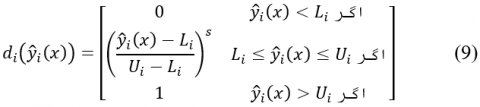## e10.png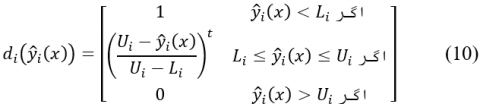To optimize the two response variables simultaneously, the total desirability function is defined concerning Eq. (11).

$D=\left(d_{1}(x) \times d_{2}(x) \times \ldots \times d_{k}(x)\right)^{\frac{1}{k}}$    (11)

6.1 Optimization case study

The present study applies the desirability function approach described above. In this approach, the desirability function for each response variable is obtained and the total desirability function is then defined.

$d(T E)=\frac{33341-(13047+1157 \times a-2800 \times b-2702 \times c-3743 \times a \times b-3486 \times a \times c-757 \times b \times c+5210 \times a \times b \times c)}{33341-4018}$

$D=[(\frac{2060-(1054.58 - 31.96×a - 234.30×b - 33.75×c- 339.26×a×b - 341.20× a×c - 350.36×b×c+ 320.48 ×a×b×c)}{2060-180})$

$×(\frac{8877-(3818.04 + 426.38×a - 745.88×b - 782.03×c- 1223.28×a×b - 1217.55×a×c- 1317.27×b×c + 1151.54×a×b×c)}{2060-180})$

$×(\frac{33341-(13047 + 1157×a - 2800 ×b - 2702 ×c - 3743 ×a×b - 3486× a×c - 757 × b× c + 5210 × a× b× c )}{33341-4018})]^\frac{1}{3}$

Table 7. Results of desirability function optimization

 a b c Results from regression D TWT TNQ TE TWT TNQ TE 9 9 9 0.9979 3.2 9.9 4194 TWT 134.5 204.5 239.5 0.9975 - - 4092 TNQ 9 9 9 0.9999 - 9.9 - TE 9 9 9 0.9999 3.2 -

To optimize the total desirability function, Lingo software was used. The results are shown in Table 7.

Each of the objective functions was optimized separately (the results are presented for comparison). The result of optimization by OptQuest software is also provided for comparison. These results were tested in the simulation model (Table 8).

As seen in Tables 7 and 8, only when the objective is to minimize the TWT, the result of optimization using Opt Quest software is better. In other cases, the result of desirability function optimization is better.

Table 8. Results of the optimization by using Opt quest, Results from Opt Quest

 a b c Results from Opt Quest TWT TNQ TE TWT 9 9 17 2.02 5.07 4431 TNQ 9 9 17 2.02 5.07 4431 TE 178 227 315 24 53 3595
7. Conclusion

The present study attempted to use a desirability function along with simulation to calculate the optimal intervals for a traffic light at an intersection. A new approach with combining the design of experiments, simulation, and desirability function was presented. Given that most of the parameters are in a state of uncertainty, to obtain the required information, Arena software was used to simulate a complex intersection. Also, we attempted to minimize the waiting time and the queue length, as well as the amount of emission at an intersection. To this purpose, first, the regression equations for each of the response variables were obtained through the design of experiments and a full-factorial design, and then a desirability function approach was applied to find the optimal amount of time for each traffic light at the intersection. Finally, the results were compared for each objective function using Arena OptQuest software.

Appendix

## a1.png## a2.png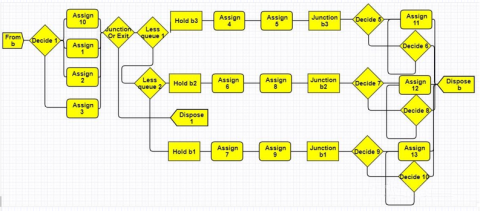## a3.pngReferences

 France, J., Ghorbani, A.A. (2003). A multiagent system for optimizing urban traffic. IEEE/WIC International Conference on Intelligent Agent Technology, IAT 2003, Halifax, NS, pp. 411-414 http://dx.doi.org/10.1109/IAT.2003.1241110

 De Schutter, B., De Moor, B. (1998). Optimal traffic light control for a single intersection. European Journal of Control, 4(3): 260-276. http://dx.doi.org/10.1016/S0947-3580(98)70119-0

 Sheu, J.B. (2006). A composite traffic flow modeling approach for incident-responsive network traffic assignment. Physica A: Statistical Mechanics and its Applications, 367: 461-478. http://dx.doi.org/10.1016/j.physa.2005.11.039

 Texas Transportation Institute, College Station, TX, “Traffic congestion and urban mobility,” [Online]. Available: http://tti.tamu.Edu/infofor/media/topics/congestionmobility.htm, accessed on 12 July 2020.

 Rossner, P., Svecova, V., Milcova, A., Lnenickova, Z., Solansky, I., Sram, R.J. (2008). Seasonal variability of oxidative stress markers in city bus drivers: Part I. Oxidative damage to DNA. Mutation Research/Fundamental and Molecular Mechanisms of Mutagenesis, 642(1-2): 14-20. http://dx.doi.org/10.1016/j.mrfmmm.2008.03.003

 Ruchirawat, M., Navasumrit, P., Settachan, D., Tuntaviroon, J., Buthbumrung, N., Sharma, S. (2005). Measurement of genotoxic air pollutant exposures in street vendors and school children in and near Bangkok. Toxicology and Applied Pharmacology, 206(2): 207-214. http://dx.doi.org/10.1016/j.taap.2004.11.025

 Brunekreef, B., Forsberg, B. (2005). Epidemiological evidence of effects of coarse airborne particles on health. European Respiratory Journal, 26(2): 309-318. http://dx.doi.org/10.1183/09031936.05.00001805

 Englert, N. (2004). Fine particles and human health—a review of epidemiological studies. Toxicology Letters, 149(1): 235-242. http://dx.doi.org/10.1016/j.toxlet.2003.12.035

 De Vasconcellos, E.A. (2005). Transport metabolism, social diversity and equity: The case of São Paulo, Brazil. Journal of Transport Geography, 13(4): 329-339. http://dx.doi.org/10.1016/j.jtrangeo.2004.10.007

 Kamrani, M., Abadi, S.M.H.E., Golroudbary, S.R. (2014). Traffic simulation of two adjacent unsignalized T-junctions during rush hours using Arena software. Simulation Modelling Practice and Theory, 49: 167-179. http://dx.doi.org/10.1016/j.simpat.2014.09.006

 Bieker, L., Krajzewicz, D., Morra, A., Michelacci, C., Cartolano, F. (2015). Traffic simulation for all: A real world traffic scenario from the city of Bologna. In Modeling Mobility with Open Data (pp. 47-60). Springer International Publishing. http://dx.doi.org/10.1007/978-3-319-15024-6_4

 Lacy, L., Gerber, W. (2004). Potential modeling and simulation applications of the web ontology language-OWL. Proceedings of the 2004 Winter Simulation Conference, Washington, DC, USA, 2004, p. 270. http://dx.doi.org/10.1109/WSC.2004.1371325

 Cornell, W.D., Cieplak, P., Bayly, C.I., Gould, I.R., Merz, K.M., Ferguson, D.M., Kollman, P.A. (1995). A second generation force field for the simulation of proteins, nucleic acids, and organic molecules. Journal of the American Chemical Society, 117(19): 5179-5197. http://dx.doi.org/10.1021/ja00124a002

 Railsback, S.F., Lytinen, S.L., Jackson, S.K. (2006). Agent-based simulation platforms: Review and development recommendations. Simulation, 82(9): 609-623. http://dx.doi.org/10.1177/0037549706073695

 Luke, S., Cioffi-Revilla, C., Panait, L., Sullivan, K., Balan, G. (2005). Mason: A multiagent simulation environment. Simulation, 81(7): 517-527. http://dx.doi.org/10.1177/0037549705058073

 Balci, O. (2004). Quality assessment, verification, and validation of modeling and simulation applications. In Proceedings of the 36th conference on Winter Simulation, Winter Simulation Conference, pp. 122-129. http://dx.doi.org/10.1109/WSC.2004.1371309

 Dalla Man, C., Raimondo, D.M., Rizza, R.A., Cobelli, C. (2007). GIM, simulation software of meal glucose-Insulin model. Journal of Diabetes Science and Technology, 1(3): 323-330. http://dx.doi.org/10.1177/193229680700100303

 Mat Tahar, R., Hussain, K. (2000). Simulation and analysis for the Kelang Container Terminal operations. Logistics Information Management, 13(1): 14-20. http://dx.doi.org/10.1108/09576050010306350

 Bush, A., Biles, W.E., DePuy, G.W. (2003). Waterway, shipping, and ports: Iterative optimization and simulation of barge traffic on an inland waterway. In Proceedings of the 35th Conference on Winter Simulation: Driving Innovation. Winter Simulation Conference, pp. 1751-1756. http://dx.doi.org/10.1109/WSC.2003.1261629

 Kesen, S.E., Baykoç, Ö.F. (2007). Simulation of automated guided vehicle (AGV) systems based on just-in-time (JIT) philosophy in a job-shop environment. Simulation Modelling Practice and Theory, 15(3): 272-284. http://dx.doi.org/10.1016/j.simpat.2006.11.002

 Chakravarthy, S.R. (2012). Reliability, health care, and simulation. Simulation Modelling Practice and Theory, 29: 44-51. http://dx.doi.org/10.1016/j.simpat.2012.07.005

 Almaz, O.A., Altiok, T. (2012). Simulation modeling of the vessel traffic in Delaware River: Impact of deepening on port performance. Simulation Modelling Practice and Theory, 22: 146-165. http://dx.doi.org/10.1016/j.simpat.2011.12.004

 Cortés, P., Muñuzuri, J., Ibáñez, J.N., Guadix, J. (2007). Simulation of freight traffic in the Seville inland port. Simulation Modelling Practice and Theory, 15(3): 256-271. http://dx.doi.org/10.1016/j.simpat.2006.11.004

 Motraghi, A., Marinov, M.V. (2012). Analysis of urban freight by rail using event based simulation. Simulation Modelling Practice and Theory, 25: 73-89. http://dx.doi.org/10.1016/j.simpat.2012.02.009

 Woroniuk, C., Marinov, M. (2013). Simulation modelling to analyse the current level of utilisation of sections along a rail route. Journal of Transport Literature, 7(2): 235-252. http://dx.doi.org/10.1590/S2238-10312013000200012

 Liu, Z. (2007). A survey of intelligence methods in urban traffic signal control. IJCSNS International Journal of Computer Science and Network Security, 7(7): 105-112.

 D’Ambrogio, A., Iazeolla, G., Pasini, L., Pieroni, A. (2009). Simulation model building of traffic intersections. Simulation Modelling Practice and Theory, 17(4): 625-640. http://dx.doi.org/10.1016/j.simpat.2008.11.001

  Ci, W.X., Ahmed, S.K., Zulkifli, F., Ramasamy, A.K. (2009). Traffic flow simulation at an unsignalized T-junction using Monte Carlo Markov Chains. 2009 IEEE International Conference on Signal and Image Processing Applications, Kuala Lumpur, 2009, pp. 346-351. http://dx.doi.org/10.1109/ICSIPA.2009.5478675

 Salimifard, K., Ansari, M. (2013). Modeling and simulation of urban traffic signals. International Journal of Modeling and Optimization, 3(2): 172. http://dx.doi.org/10.7763/IJMO.2013.V3.261

 https://en.wikipedia.org/wiki/European_emission_standards#cite_note-nytimes_23sep2015-14, accessed on 3 November 2020.

 Bhutta, K.S., Huq, F. (2002). Supplier selection problem: A comparison of the total cost of ownership and analytic hierarchy process approaches. Supply Chain Management: An International Journal, 7(3): 126-135. http://dx.doi.org/10.1108/13598540210436586

 Candioti, L.V., De Zan, M.M., Cámara, M.S., Goicoechea, H.C. (2014). Experimental design and multiple response optimization. Using the desirability function in analytical methods development. Talanta, 124: 123-138. http://dx.doi.org/10.1016/j.talanta.2014.01.034